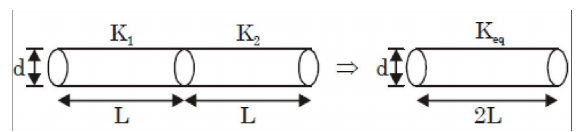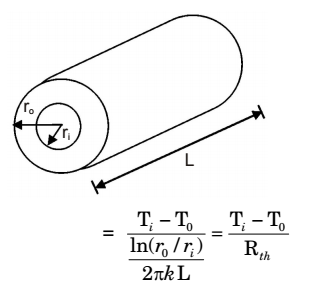## Heat Transfer Miscellaneous

#### Heat-Transfer

Direction: Water (specifie heat, c = 4.18 kJ/kgK) enters a pipe at a rate 0.01 kg/s and a temperature of 20°C. The pipe, of diameter 50 mm and length 3 m, is subjected to a wall heat flux q"w in W/m2.

1. If q"w = 5000 and the convection heat transfer coefficient at the pipe outlet is 1000 W/m2K, the temperature in °C at the inner surface of the pipe at the outlet is

1. q"w = 5000 w/m2
h = 1000 w/m2k
Heat transfer through pipe wall, Q = q"w × surface area
= 5000 × π × d × l
= 5000 × 3.14 × 0.05 × 3 = 2355 W
Q = mCP (T0 –Ti), amount of heat gained by the water
2355 = 0.01 × 4180 (T0 – 20)
T0 = 76.33°Cq"w = h( Tso - To)
5000 = 1000 (Tso – 76.33)
Tso = 81°C

##### Correct Option: D

q"w = 5000 w/m2
h = 1000 w/m2k
Heat transfer through pipe wall, Q = q"w × surface area
= 5000 × π × d × l
= 5000 × 3.14 × 0.05 × 3 = 2355 W
Q = mCP (T0 –Ti), amount of heat gained by the water
2355 = 0.01 × 4180 (T0 – 20)
T0 = 76.33°Cq"w = h( Tso - To)
5000 = 1000 (Tso – 76.33)
Tso = 81°C

1. If q"w = 2500 x, where x is in m and in the direction of flow (x = 0 at the inlet), the bulk means temperature of the water leaving the pipe in °C is

1. At the inlet of pipe
x = O
q "wi = 2500 × 0 = 0
At the exit of pipe,
x = l

 q "wi = 2500 × 1 × 3 = 7500 W m2

Average heat transfer,
 q "avg q "wi + q "we = 0 + 7500 = 3750 w 2 2 m2

Net heat transfer
Q = q "avg × surface area = 3750 × πdl
= 3750 × 3.14 × 0.05 × 3 = 1766.25 W
Also Q = mCp (T0 – Ti)
1766.25 = 0.01× 4180 (T0 –20)
By solving above equation, we get
T0 = 62° C

##### Correct Option: B

At the inlet of pipe
x = O
q "wi = 2500 × 0 = 0
At the exit of pipe,
x = l

 q "wi = 2500 × 1 × 3 = 7500 W m2

Average heat transfer,
 q "avg q "wi + q "we = 0 + 7500 = 3750 w 2 2 m2

Net heat transfer
Q = q "avg × surface area = 3750 × πdl
= 3750 × 3.14 × 0.05 × 3 = 1766.25 W
Also Q = mCp (T0 – Ti)
1766.25 = 0.01× 4180 (T0 –20)
By solving above equation, we get
T0 = 62° C

1. A slender rod of length L, diameter d (L >> d) and thermal conductivity k1 i s joined with another rod of identical dimensions, but of thermal conductivity k 2, to form a composite cylindrical rod of length 2L. The heat transfer in radial direction and contact resistance are negligible. The effective thermal conductivity of the composite rod is

1.As per question, heat transfer in radial direction and contact resistance are negligible. In series connection,
(Rth) eq = R1 + R2

 2L = L + L Akeq AK1 AK2

 2 = 1 + 1 Keq K1 K2

 ⇒ Req = 2K1K2 K1 + K2

##### Correct Option: AAs per question, heat transfer in radial direction and contact resistance are negligible. In series connection,
(Rth) eq = R1 + R2

 2L = L + L Akeq AK1 AK2

 2 = 1 + 1 Keq K1 K2

 ⇒ Req = 2K1K2 K1 + K2

1. A hollow cylinder has length L, inner radius r1 outer radius r2, and thermal conductivity k. The thermal resistance of the cylinder for radial conduction is

1.  In(r2/r1) 2πkL

##### Correct Option: A

 In(r2/r1) 2πkL

1. Consider a long cylindrical tube of inner and outer radii, ri and r0, respectively, length L and thermal conductivity, k. Its inner and outer surfaces are maintained at Ti, and T0, respectively (Ti > T0). Assuming one dimensional steady state heat conduction in the radial direction, the thermal resistance in the wall of the tube is

1. Ar = 2πrL
From Fourier ’s Law

 qr = kAr dT dr

 qr = 2π krL dT dr

Boundary conditions:
T = Ti at r = ri
T = T0 at r = r0
 q = 2πkL(Ti - T0) In(r0/ri)Rth = In(r0/ri) 2πkL

##### Correct Option: C

Ar = 2πrL
From Fourier ’s Law

 qr = kAr dT dr

 qr = 2π krL dT dr

Boundary conditions:
T = Ti at r = ri
T = T0 at r = r0
 q = 2πkL(Ti - T0) In(r0/ri)Rth = In(r0/ri) 2πkL